# Arithmetic average

The arithmetic mean of the five numbers is exactly 8. The sum of these four numbers is 30. What is the fifth number?

x =  10

### Step-by-step explanation:

(30+x)/5 = 8

x = 10

Our simple equation calculator calculates it.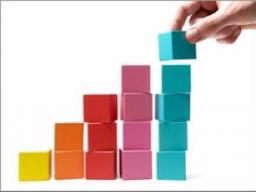Did you find an error or inaccuracy? Feel free to write us. Thank you!Tips to related online calculators
Looking for help with calculating arithmetic mean?
Looking for a statistical calculator?
Do you have a linear equation or system of equations and looking for its solution? Or do you have a quadratic equation?

#### You need to know the following knowledge to solve this word math problem:

We encourage you to watch this tutorial video on this math problem:

## Related math problems and questions:

• The average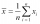The average of one set of 4 numbers is 35. The average of another set of number is 20. The average of the numbers in the two sets is 30. How many numbers are there in the other set?
• Classs meanClass size is 30 students, math was not worse than sign 2. Determine the number of pupils who were sign 1 if the class had a 1.4 sign average.
• Digit sumFind the smallest natural number n, the digit sum of which is equal to 37.
• Certificate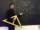There is 31 students in a class. From mathematics was'nt worse mark than 2. Average mark in mathematics was 2. How many students have mark 1 and how many mark 2?
• Two friends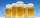Two friends met as a good man perish together for a beer. After recovery the most important topics (politics, women, football ...), one asks: - And how many do you have children? - I have 3 children. - And how many years have? Friend already not want to a
• The sum 12The sum of 3 mixed numbers is 20 13/15. two of the numbers are 6 1/3 and 7 5/6. what is the third number?
• Even / odd numbers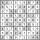a / Using variable n write two consecutive odd numbers. b / the sum of three consecutive odd numbers is 333. What are this numbers?
• Unknown numberSamuel wrote unknown number. Then he had add 200000 to the number and the result multiply by three. When it calculated he was surprised, because the result would have received anyway, if write digit to the end of original number. Find unknown number.
• TranslateTranslate the following mathematical statement into an algebraic expression or equation. 1. Nine less that the quotient of a number and 3 2. The ratio of 3 and the sum of 4 and an unknown number 3. Eighty is the product of 4 and g 4. The sum of x and 18 i
• Sum and roundingI know two numbers whose sum is 20. When they each round and then added together I get the 30. What are this numbers?
• One-thirdA one-third of unknown number is equal to five times as great as the difference of the same unknown number and number 28. Determine the unknown number.
• 7 digit numberIf 3c54d10 is divisible by 330, what is the sum of c and d?
• Taken away from number18 is taken away from 8 times of a number is 30. Find the number.
• NumbersDetermine the number of all positive integers less than 4183444 if each is divisible by 29, 7, 17. What is its sum?
• Find the meanFind the number between 13 and 29.
• The sumThe sum of five consecutive odd numbers is 75. Find out the sum of the second and fourth of them.
• NumberI think the number. If I add to its third seven I get same as when to its quarter add 8. Which is the number?